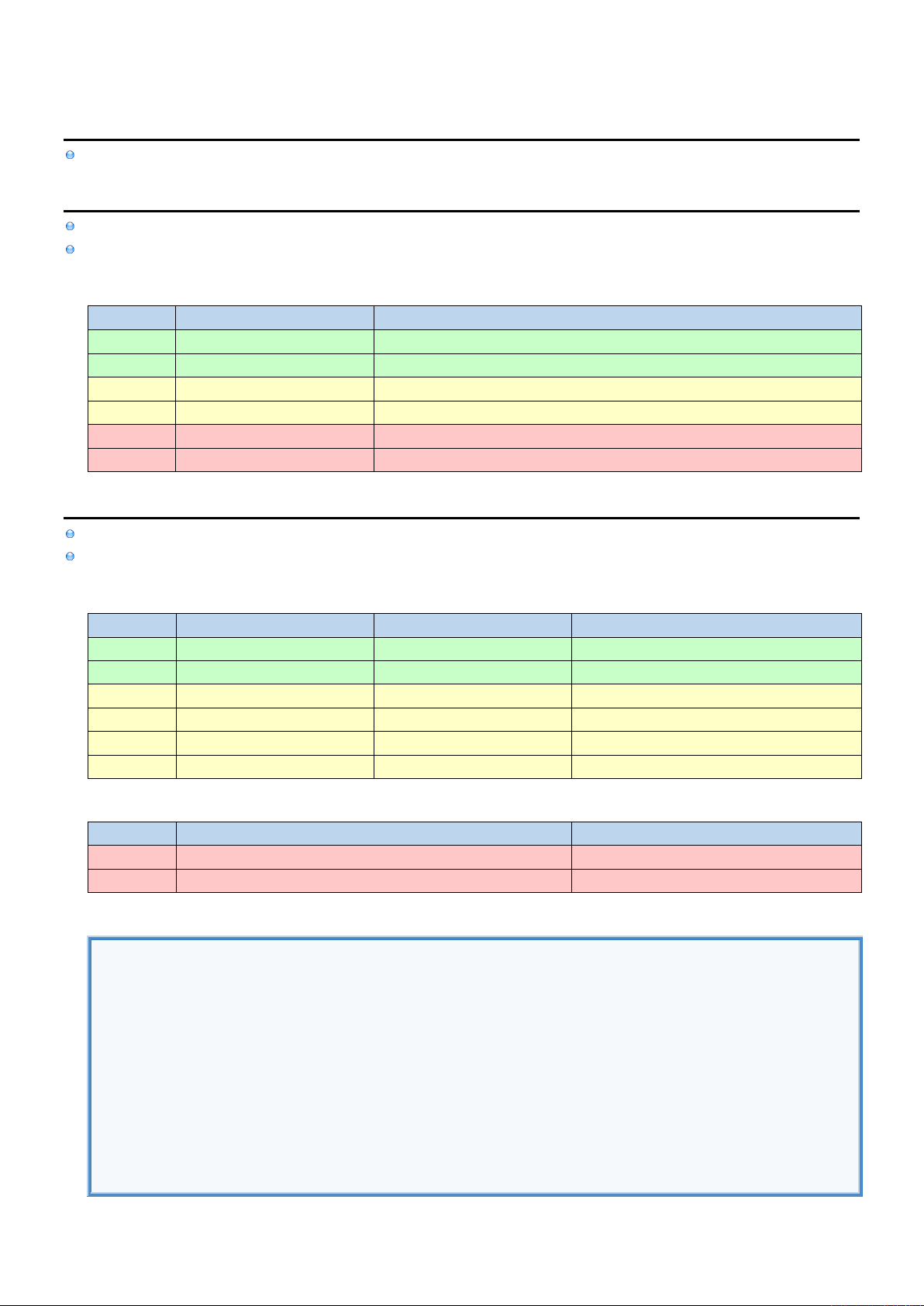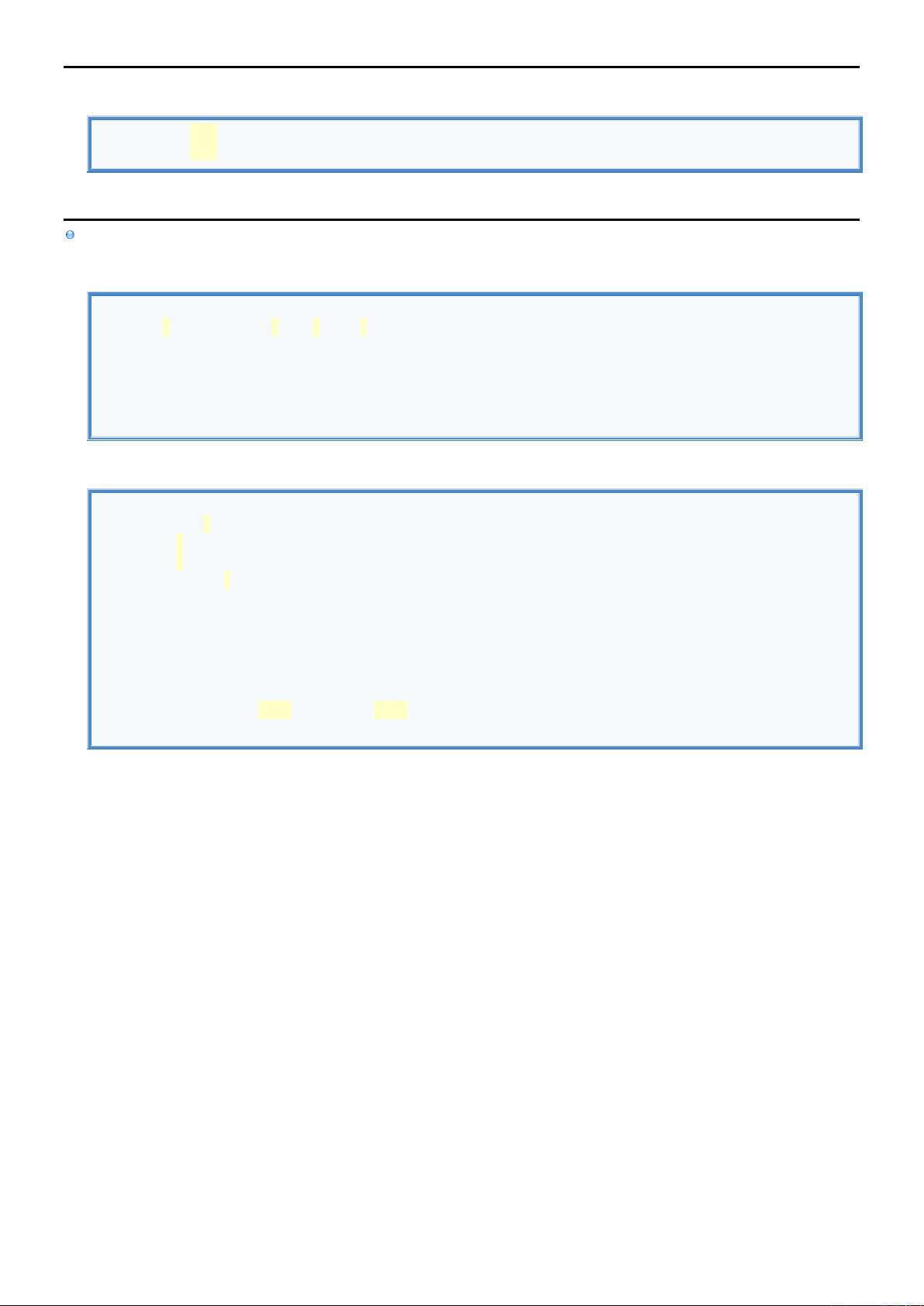3
3
.
.
2
2
D
D
a
a
t
t
a
a
T
T
y
y
p
p
e
e
s
s
I
I
n
n
f
f
o
o
Int/UInt are aliases for Int32/UInt32 or Int64/ UInt64 (depending if the platform is 32-bit or 64-bit respectively).
L
L
i
i
t
t
e
e
r
r
a
a
l
l
s
s
Literal is string representation of both data type and data value: 10, "John", ["AUDI", "FIAT"]
Literal notations are different ways of constructing literals that represent the same data type: 10, +10, 012 or 0xA.
Literals
LITERAL
EXAMPLE
NOTATIONS
Nil
nil
Boolean
true, false
Integer
65
Decimal: 65 Hexadecimal: 0x41 Octal: 0o101 Binary: 0b1000001
Float
65.4
Basic: 65.4 Scientific: 0.654E+2
String
"Hello \(name) \n"
Array
["John", "Bill"]
D
D
a
a
t
t
a
a
T
T
y
y
p
p
e
e
s
s
Swift has no distinction between primitive data types (like int in java) and non-primitive data types (like String in java).
All of the below Data Types are implemented as Structures.
Scalar Data Types
TYPE
EXAMPLE
DESCRIPTION
TYPES / SIZE
Nil
var value : Int? = nil
undefined/unknown value
Bool
var value : Bool = true
logical TRUE or FALSE
Int
var value : Int = -65
Signed integer
Int, Int8, Int16, Int32, Int64
UInt
var value : UInt = 65
Unsigned integer
UInt, UInt8, UInt16, UInt32, UInt64
Float
var value : Float = 65.4
Real number
32-bit IEEE 754
Double
var value : Double = 65.4
Real number
64-bit IEEE 754
Collection Data Types
TYPE
DESCRIPTION
String
Class for storing constant strings.
Array
Array of Strings
Data Types & Literals
//GENERAL
var value1 : Int? = nil //Nil Literal
var value2 : Bool = true //Boolean Literal: true, false
//NUMBERS
var value3 : Int = -65 //Integer Literal: 0x41 0o101 0b1000001
var value4 : UInt = 65 //Integer Literal: 0x41 0o101 0b1000001
var value5 : Float = 65.4 //Float Literal: 0.654E+2
var value6 : Double = 65.4 //Float Literal: 0.654E+2
//COLLECTIONS
var value7 : String = "Hello \(name) \n" //String
var value8 : [String] = ["John", "Bill"] //ArrayD
D
a
a
t
t
a
a
T
T
y
y
p
p
e
e
A
A
l
l
i
i
a
a
s
s
Data Type Alias
typealias name = String //DECLARE ALIAS.
var person : name = "John" //USE ALIAS.
G
G
e
e
n
n
e
e
r
r
i
i
c
c
D
D
a
a
t
t
a
a
T
T
y
y
p
p
e
e
s
s
After the name define list of Generic Data Types.
Generic Function (with Numeric Constraint)
//DECLARE GENERIC FUNCTION.
func add<T: Numeric> (x: T, y: T) -> (T) { return x + y }
//USE FUNCTION
var result1 = add(x: 10, y:20) //Use Integeres
var result2 : Float = add(x: 10.4, y:20.4) //Use Floats
print(result1)
Generic Struct (with Numeric Constraint)
//DECLARE GENERIC STRUCT.
struct Numbers<T: Numeric> {
var x : T
var y : T
func add () -> (T) { return x + y }
}
//USE STRUCT.
var numbers1 = Numbers(x: 10, y: 20) //Use Integeres